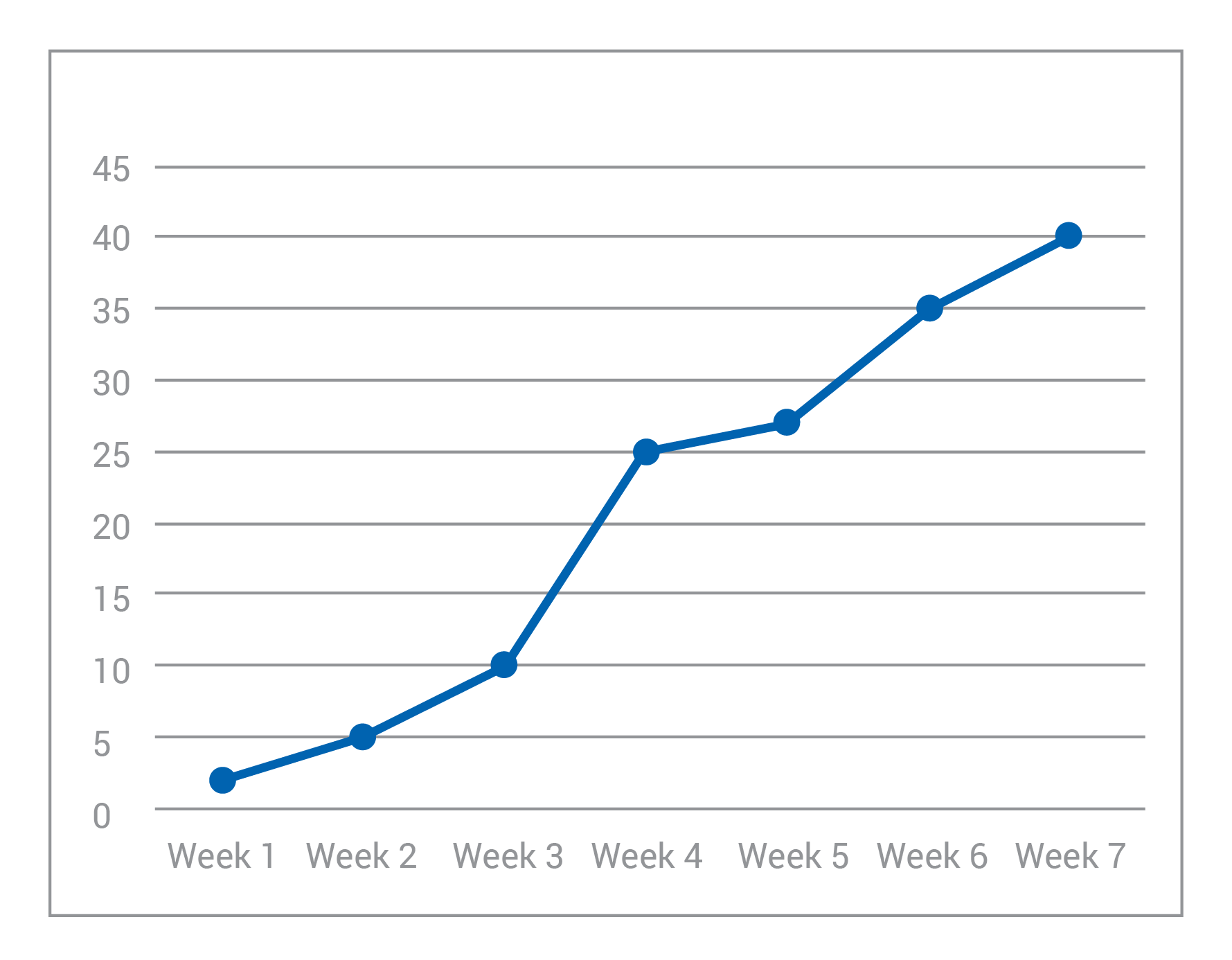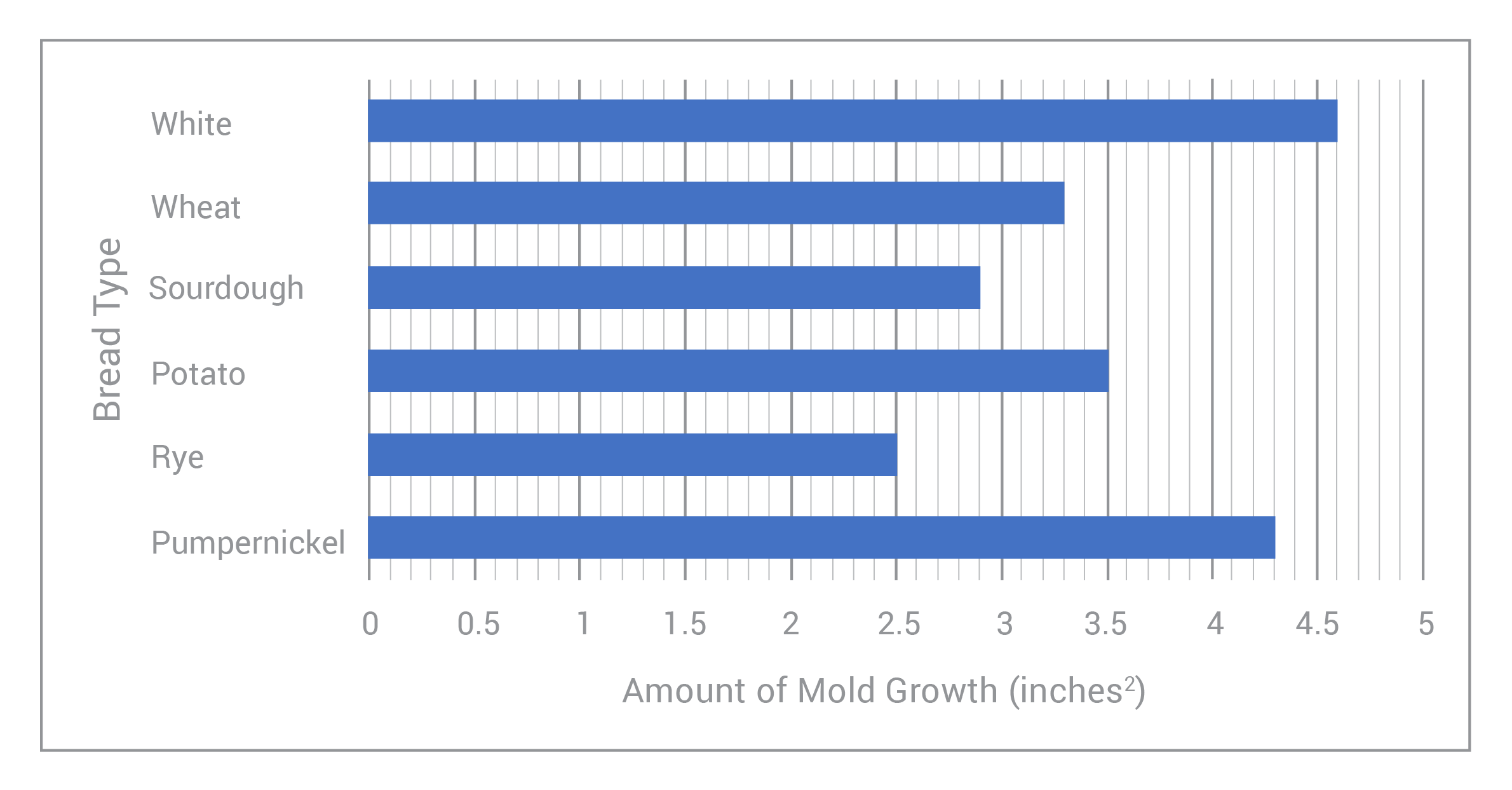# Identifying Independent and Dependent VariablesHey guys! Welcome to this Mometrix video over Independent and Dependent Variables.

There are two primary variables in a scientific experiment: the independent and dependent variable.

## What is an independent variable?

The independent variable is the variable that is controlled throughout the experiment, but it is not affected by any other variable. So, like its name suggests, it is independent from other variables. Time, for example, will always be an independent variable. Let’s say you wanted to test how tall a tree would grow after eight months of treating it with a certain type of fertilizer. The height of the tree may change, and you can change the amount of fertilizer, but that will have no effect on the eight month period. You can never speed up time or slow time down. It will always be independent from anything we can do. However, you can change the amount of time you are measuring something.

## What is a dependent variable?

The dependent variable is the variable being tested. The dependent variable depends on other factors. In our last example we said, “how tall will a tree grow after eight months of treating it with a certain type of fertilizer?” Well, the height of the tree is our dependent variable. It is dependent on the amount of time, the type of fertilizer, as well as other things like the region it’s growing in, temperature, and so on.

It may be helpful to think of the independent variable and dependent variable in the same vein as cause and effect. If the independent variable is changed, then an effect will be observed in the dependent variable.

Both independent and dependent variables may change during the duration of the experiment. However, the experimenter is in control of changing the independent variable but not the dependent variable. The dependent variable solely changes under the influence of the independent variable.

When graphing it is sort of a consensus that you would always use the x-axis to plot the independent variable and the y-axis to plot the dependent variable. However, some people don’t, and that is why it is so important to label your axes!

I hope that this video was helpful! If you enjoyed it, then be sure to give us a thumbs up, and subscribe to our channel for further videos.

See ya guys next time!

## Practice Questions

Question #1:

Carlos is developing a new chocolate chip recipe. While testing recipes out, he notices that when he adds less and less flour to the cookie dough, the cookies get wider and spread out on the pan. What is the dependent variable in Carlos’ experiment?

Type of flour used

Remember that the dependent variable depends on the independent variable. In this problem, we see that the width (a.k.a. the “size”) of each cookie depends on how much flour Carlos puts in the dough.

*Another helpful rule to memorize is: the dependent variable is what we observe (or measure) happening in an experiment. But, as for the independent variable, it is usually what we change in an experiment. In this problem, the independent variable is the amount of flour that Carlos adds to the dough.

Question #2:

A local gardening club has started a new compost bin, and they begin by putting a small number of worms in the starter soil. Every week, they open the bin and count how many worms are working through the compost. Using this information and the graph below, choose which measurement is the independent variable.Time (weeks passed)

Length of worms in the compost bin

Amount of worms in the compost bin

Amount of compost generated

The correct answer is A. While we may not be making time go on or changing time itself, we see that the amount of worms grows as time goes on. Hence: the worm population depends on how much time has passed.

*Also, even if we didn’t fully understand what was going on in this experiment, we can count on two “cheat cards:” time is almost always an independent variable; and we usually put the independent variable on the x-axis and the dependent variable on the y-axis when graphing experiment results.

Question #3:

You’ve been given the equation $$q=7.4p^2+9$$ and the table:

 (input)p 2 4 6 8 10 12 14 16 (output)q 39 129 279

Using this information, we can deduce that the dependent variable is most likely _____.

$$p$$
$$7.5$$
$$q$$
$$p^2$$

The correct answer is C. If we were only given the equation $$q=7.4p^2+9$$, we couldn’t decide which variable depends on the other (because we could easily re-write the equation as $$p=\sqrt{\frac{q-9}{7.4}}$$). However, because we know that p is the input-value and q is the output-value, we also know that q depends on p. (What we pull out depends on what we put into the equation.) Also, since we’ve already been given pre-set values of p, it’s safe to assume that p is the independent variable; because the value of p will not be impacted by a change in q.

Question #4:

Over the past few weeks, Briana has been conducting an experiment to see what type of bread grows the most mold within a given period of time. She’s chosen 6 different types of bread, and as time has passed, she’s measured the areas of each mold patch that’s grown on the respective bread slices. The graph below shows her results:What is the independent variable in Briana’s experiment?

Time (weeks passed)

Amount of mold growth (inches2)

The correct answer is B. While it may be tempting to choose Choice A, time is actually a controlled variable (the “control”) in this experiment. We observed a phenomenon over a pre-set, controlled period of time. Really, we need to ask ourselves, “What is Briana changing?” (this will always be our independent variable) and “What is she measuring/observing?” Of course, as Briana has changed the type of bread, she’s observed a change in the amount of mold grown on each slice; so it’s clear that our independent variable is the bread type!

*Additionally, Briana has noticed that the amount of mold growth on each slice is different for each type of bread. Hence, it’s safe to say that the ‘amount of mold growth’ depends on the ‘bread type.’ Meaning: the amount of mold growth is Briana’s dependent variable!

**One final note: notice that the dependent variable sits on the x-axis of this graph, and the independent variable sits on the y-axis. This is different from what we usually see! So, it’s important that we take the “x-axis=independent variable and y-axis=dependent variable” trick with a grain of salt. Always read the question and the graph labels carefully!

Question #5:

Mia is training for a marathon. As she reads through her food & fitness tracking journal, she notices that she runs more miles on the days where she eats an apple with breakfast. What is the dependent variable in this scenario?

Amount of miles Mia runs each day

What Mia eats for breakfast

Amount of miles Mia will run in the marathon

When Mia eats an apple

The correct answer is A. In this problem, the amount of miles that Mia runs in a day depends on whether or not she eats an apple with her breakfast. We can also note that the independent variable is what Mia eats for breakfast. More specifically, it is whether or not she eats an apple with her breakfast.